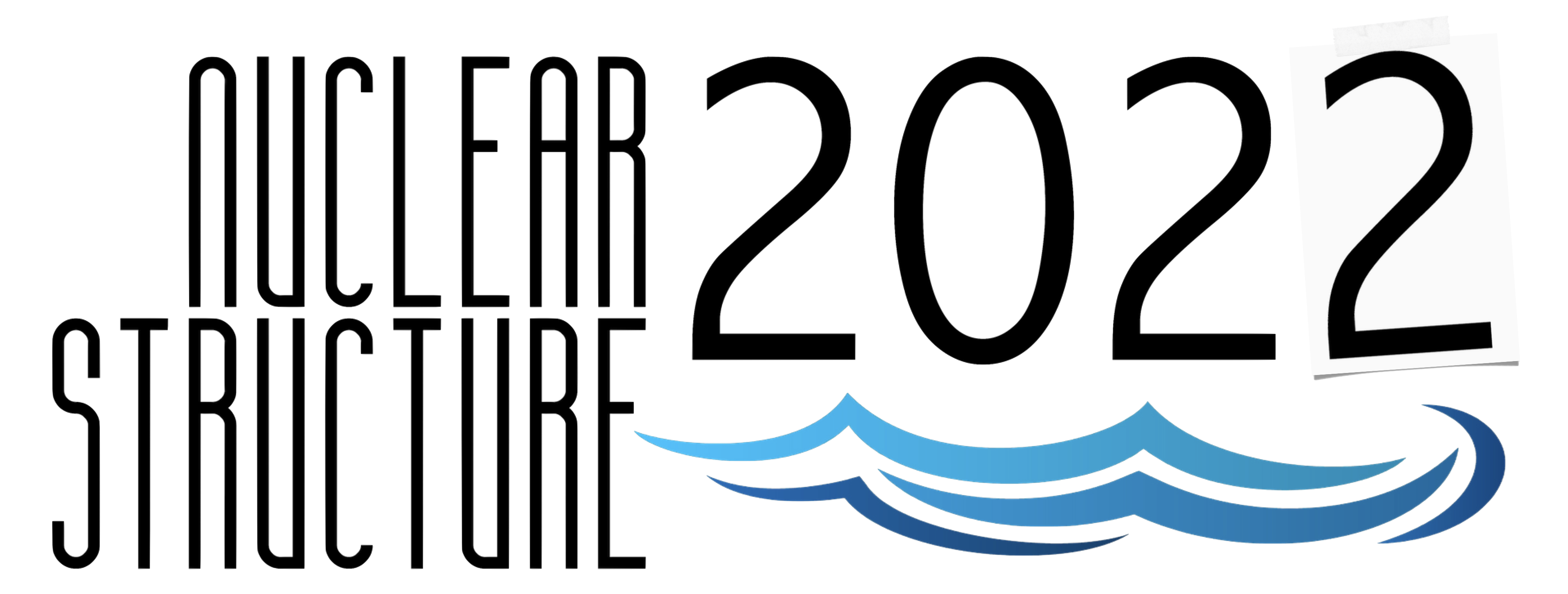#Nuclear Structure 2022

Jun 13 – 17, 2022
Berkeley, CA
US/Pacific timezone

## The low-lying structure of $^{211}$At

Not scheduled
1m
Berkeley, CA

#### Berkeley, CA

Lawrence Berkeley National Laboratory
Oral Poster Presentations

### Speaker

Vasil Karayonchev (Institute for Nuclear Physics, University of Cologne, TRIUMF)

### Description

Lifetimes of low-energy states in $^{211}$At have been measured using the recoil-distance Doppler shift, Doppler-shift attenuation, and fast-timing methods at the University of Cologne. The obtained reduced transition probabilities have been compared to two shell-model calculations, a large-scale shell-model calculation using the Kuo-Herling residual interaction and a calculation using a single-$j$ approximation for protons in the $0h_{9/2}$ orbital. The large-scale calculations, which account only for single particle-hole excitations, significantly overestimate some of the ground-state transition probabilities, especially the $B(E2;13/2^-_1 \rightarrow 9/2^-_1)$ value. This discrepancy has been attributed to the presence of higher-order particle-hole excitations in the wave function of the ground state, which are not accounted for by the Kuo-Herling intaraction. The effects of those excitations on the transition rates, however, are weaker in $^{211}$At than they are in $^{210}$Po. A modification to the $(h_{9/2},h_{9/2}| \hat{V} |h_{9/2},f_{7/2})_{J=2}$ two-body matrix element has been introduced which leads to a considerably better description of the structure of $^{210}$Po and $^{211}$At. However, the origin of this effect needs to be further investigated. The newly obtained reduced transition probabilities are described very well by a single-$j$ calculation. This, together with the fact that the energy spectrum of $^{211}$At is also well described, indicates that seniority can be regarded as a good quantum number in $^{211}$At. It would be of interest to continue the same study along heavier $N=126$ isotones, where information on most $E2$ transitions in even-even and odd-even nuclei is still missing.

### Primary authors

Vasil Karayonchev (Institute for Nuclear Physics, University of Cologne, TRIUMF) Prof. J. Jolie (Institute for Nuclear Physics, University of Cologne) A. Blazhev (Institute for Nuclear Physics, University of Cologne) C. Fransen (Institute for Nuclear Physics, University of Cologne) A. Esmaylzadeh (Institute for Nuclear Physics, University of Cologne) L. Knafla (Institute for Nuclear Physics, University of Cologne) P. Van Isacker (Grand Accelerateur National d'Ions Lourds, CEA/DRF--CNRS/IN2P3)

### Presentation materials

There are no materials yet.